407 493 6601

# 7th Grade - Complementary & Supplementary Angles

## Introduction

• The sum of two angles defines complementary and supplementary angles.

## Complementary Angles

• Two acute angles form complementary angles.
• The sum of these two angle measures is 90o (right angle).
• Each angle complements the other.
• In the diagram shown below, , and hence they are complementary angles.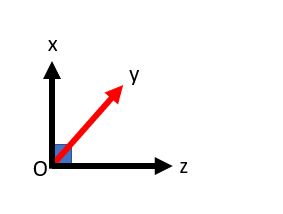• Complementary angles do not have to be always adjacent angles. For example,  shown below are not adjacent angles, but still, they are complementary angles.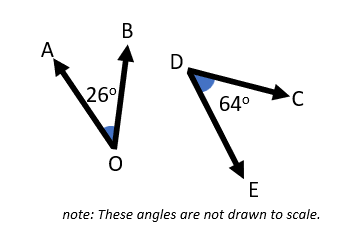## Supplementary Angles

• Two angles are said to be supplementary if their sum measures $180°$.
• In other words, two angles that form a line are called supplementary angles.
• Each angle supplements the other angle.
• In the diagram shown below, , and hence they are a pair of supplementary angles.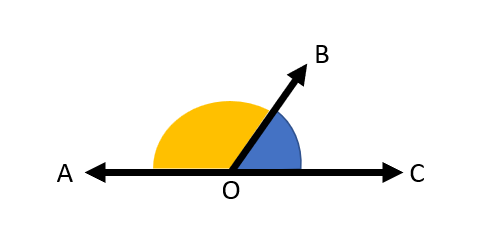• Supplementary angles do not have to be always adjacent angles. For example,  shown below are not adjacent angles, but still, they are supplementary angles.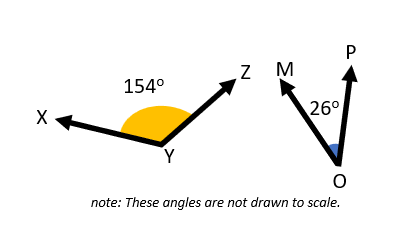## Solved Examples

Question1: Find the measures of $\angle ABC$ and $\angle XYZ$ if they are complementary.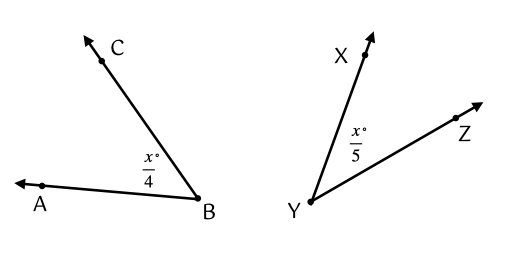Solution: The sum of two complementary angles is $90°$.

$\angle ABC+\angle XYZ=90°$

$\frac{x}{4}+\frac{x}{5}=90°$

$\frac{9x}{20}=90°$

$x=\frac{90°×20}{9}$

$x=200°$

$\angle ABC=\frac{x}{4}$$=\frac{200°}{4}$$=50°$

$\angle XYZ=\frac{x}{5}$$=\frac{200°}{5}$$=40°$

Question 2: The difference between two supplementary angles is 20 degrees. Find the measure of both angles.

Solution: Let one of the angles be $x°$. Then, the other angle will be $\left(180-x\right)°$.

According to the question:

$\left(180-x\right)°-x°=20°$

$180°-2x°=20°$

$2x°=180°-20°$

$2x°=160°$

$x°=\frac{160°}{2}$$=80°$

So, one angle is $80°$. Then, the other angle will be $100°$.

## Cheat Sheet

• Complementary Angles:
• Two angles are said to be complementary if their sum is $90°$.
• The complement of an angle $x°$ is $\left(90-x\right)°$.
• Supplementary Angles:
• Two angles are said to be supplementary if their sum is $180°$.
• The supplement of an angle $y°$ is $\left(180-y\right)°$.

## Blunder Areas

• Two angles that add up to $90°$ are complementary angles. Both angles are not necessarily adjacent.
• Two angles that add up to $180°$ are supplementary angles. Both angles are not necessarily adjacent.